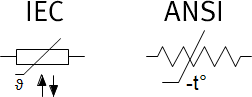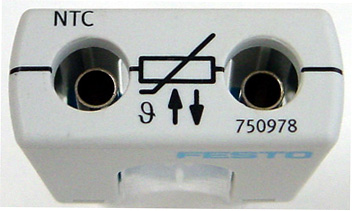# Resistor, temperature-dependent (NTC)In a temperature-dependent resistor of type NTC (Negative Temperature Coefficient), the resistance value falls as the temperature rises.

Temperature-depended resistors can be heated by external or internal heating. In case of external heating, the component is heated by ambient temperature and by the current running through the component.

The local ambient temperature T_e can be changed during simulation and is displayed as a colored scale with a pointer. The arising component temperature T is not shown symbolically. But it can be shown in a diagram or the circuit.

A Steinhart-Hart-equation with four coefficients is used as mathematical model. The coefficients are automatically calculated by FluidSIM by providing four reference resistance values R1 - R4.